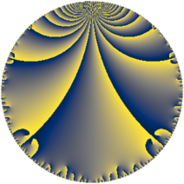# Properties

 Label 75.6.iLevel $75$ Weight $6$ Character orbit 75.i Rep. character $\chi_{75}(4,\cdot)$ Character field $\Q(\zeta_{10})$ Dimension $96$ Newform subspaces $1$ Sturm bound $60$ Trace bound $0$

# Learn more about

## Defining parameters

 Level: $$N$$ $$=$$ $$75 = 3 \cdot 5^{2}$$ Weight: $$k$$ $$=$$ $$6$$ Character orbit: $$[\chi]$$ $$=$$ 75.i (of order $$10$$ and degree $$4$$) Character conductor: $$\operatorname{cond}(\chi)$$ $$=$$ $$25$$ Character field: $$\Q(\zeta_{10})$$ Newform subspaces: $$1$$ Sturm bound: $$60$$ Trace bound: $$0$$

## Dimensions

The following table gives the dimensions of various subspaces of $$M_{6}(75, [\chi])$$.

Total New Old
Modular forms 208 96 112
Cusp forms 192 96 96
Eisenstein series 16 0 16

## Trace form

 $$96q + 352q^{4} - 120q^{5} + 72q^{6} - 1230q^{8} + 1944q^{9} + O(q^{10})$$ $$96q + 352q^{4} - 120q^{5} + 72q^{6} - 1230q^{8} + 1944q^{9} - 890q^{10} + 474q^{11} + 2748q^{14} - 780q^{16} + 1910q^{17} - 5522q^{19} - 19220q^{20} + 1764q^{21} + 6170q^{22} + 21980q^{23} + 13824q^{24} + 11790q^{25} - 11028q^{26} - 9120q^{28} - 24304q^{29} - 22860q^{30} + 1566q^{31} - 3690q^{33} + 24224q^{34} + 10250q^{35} - 28512q^{36} + 16490q^{37} + 68730q^{38} - 12168q^{39} - 106480q^{40} + 26126q^{41} - 43560q^{42} - 10814q^{44} + 9720q^{45} + 22956q^{46} + 66440q^{47} - 123480q^{49} + 329620q^{50} - 83232q^{51} + 251240q^{52} - 91690q^{53} - 5832q^{54} - 123470q^{55} + 116100q^{56} - 483290q^{58} - 97428q^{59} + 119610q^{60} + 91560q^{61} - 138410q^{62} + 47790q^{63} + 127738q^{64} - 235130q^{65} + 118296q^{66} + 163960q^{67} - 73332q^{69} + 209520q^{70} + 155672q^{71} - 99630q^{72} + 18980q^{73} + 100588q^{74} - 464032q^{76} + 119560q^{77} - 112020q^{79} - 66300q^{80} - 157464q^{81} + 148010q^{83} - 61128q^{84} + 346980q^{85} - 295956q^{86} - 371160q^{87} - 189090q^{88} - 494082q^{89} - 144180q^{90} - 79494q^{91} + 599810q^{92} + 234622q^{94} + 605800q^{95} + 210744q^{96} + 372140q^{97} + 891060q^{98} + 25596q^{99} + O(q^{100})$$

## Decomposition of $$S_{6}^{\mathrm{new}}(75, [\chi])$$ into newform subspaces

Label Dim. $$A$$ Field CM Traces $q$-expansion
$$a_2$$ $$a_3$$ $$a_5$$ $$a_7$$
75.6.i.a $$96$$ $$12.029$$ None $$0$$ $$0$$ $$-120$$ $$0$$

## Decomposition of $$S_{6}^{\mathrm{old}}(75, [\chi])$$ into lower level spaces

$$S_{6}^{\mathrm{old}}(75, [\chi]) \cong$$ $$S_{6}^{\mathrm{new}}(25, [\chi])$$$$^{\oplus 2}$$